## Annotation

If you take any sum, then all other sums can be divided into three groups: identical sums, decomposition sums, and other sums. The classification criteria are the terms and the result of the addition.

## Identical sums

Identical sums are combined into a separate group by the commutativity property. Sums from this group have pairwise identical terms and an equal number of terms. The result of adding these sums is the same. Here is an example of identical sums.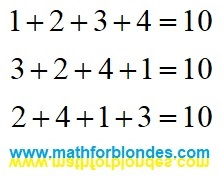Identical sums

The number of sums in this group is determined by the number of terms. For infinite sums, it is equal to infinity.

## Decomposition sums

If the results of adding a different number of terms or terms of different sizes are the same, then such sums form a decomposition group.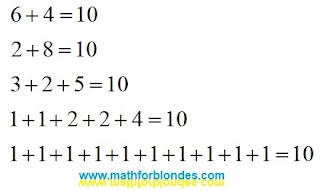Decomposition sums

Any of these sums can be obtained by decomposing the result of addition into terms using linear angular functions. Having thus obtained two terms, any of them can also be decomposed into terms, and so on. An example expansion for the first three sums: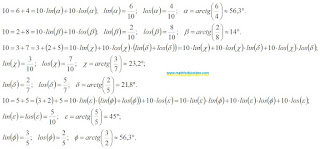Decomposing into terms

An example of decomposing a number into three terms shows that different decomposition options can give the same sum, which underlies the associative properties of addition. The more terms the sum contains, the more different expansion options there can be. The expansion into terms can be continued indefinitely. Different angles and different decomposition algorithms make it possible to obtain different variants of infinite sums. The theory of limits allows you to determine the result of addition based on the analysis of terms. The expansion into terms allows the summation result to be represented as an infinite series of terms.

For example, let's decompose the unit into an infinite sum according to the following principle: the expansion is performed at an angle of 45°, each second term is decomposed into two terms.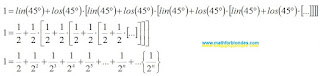Decomposition into an infinite number of terms

The curly brackets indicate the sum of the invisible compensating group of terms, which complements the result of addition to an integer unit. It can be assumed that any sum, even an infinite divergent series, in trigonometric form is equal to one.

## Other sums

If the result of adding any amount differs from the result of adding the amount in question, then this amount has nothing to do with the amount in question and belongs to the “other sums” group.

Let's assume that for the sum a+b=c there is another addition result d that is not equal to c. That is, a+b=d. We represent these two expressions using linear angle functions, and then we translate them into a trigonometric form: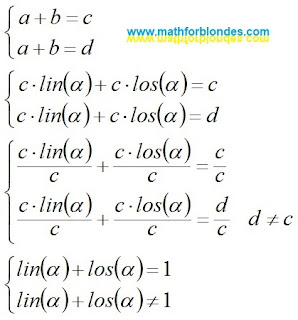Different addition results

The assumption that there are different addition results for the same sum takes us beyond the boundaries of mathematics, where the basic trigonometric relations stop working: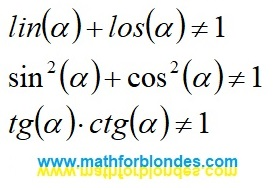Beyond the boundaries of mathematics

Why can't the same sum of terms have two different addition results? You can understand this by considering the reverse process - the transformation of trigonometric functions into specific mathematical sums. I will cover this in more detail in a separate post.

In conclusion of the conversation about the presence of two different results of addition in divergent series, I will give an example from physics. In the earth's crust (convergent series) there are natural caves (the sum of the convergent series). Using special mechanisms (convergence according to Cesaro, etc.) we can get artificial tunnels (the sum of a convergent series). There are no natural caves in the seas and oceans (divergent series, the sum of the series is missing). The use of special mechanisms (convergence according to Cesaro, etc.) allows us to obtain artificial tunnels (the sum of a divergent series) in the water column. Based on this mathematically proven theory, it is possible to design a network of tunnels for roads and railways that encircle the entire earth's surface. Such a theory is quite possible if we do not understand the difference between solids (convergent series) and liquids (divergent series).

## Conclusion

There are no mathematical methods that allow you to get a different addition result for the sum in question.

## Annotation

The use of a shift in arithmetic leads to the addition of terms with different units of measurement.

Consider the results of a shift in arithmetic addition. A shift is possible when performing addition in a column. For example, let's take a three-digit number and add to it the same number without a shift, with a shift by one position and with a shift by two positions. At the same time, we will observe the rule adopted when shifting infinite series - we discard the numbers of the second term that go beyond the boundaries of the first number.Addition with a shift in a column

Shifting by a different number of positions leads to different results in the same way as when shifting infinite series. You can write the same expressions in a line with the subsequent indication of the units of measurement for each of the terms.Addition with a shift in a line

## Conclusion

The shift leads to a change in the unit of measurement of the shifted term. The shift is a violation of the basic law of addition - you cannot add terms with different units of measurement.

## Trigonometry value table for children

In trigonometry value table lists the sines, cosines, and tangents of multiples of 15 degrees from 0 to 90 degrees.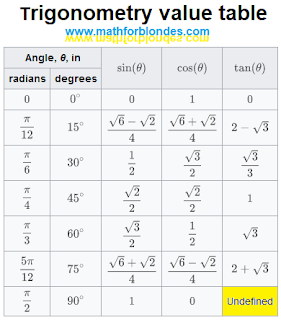Trigonometry value table

Why is this table of trigonometry values for kids (and mathematicians)? Firstly, the values of trigonometric functions are not given in numerical form, but in the form of mathematical expressions using square roots. Secondly, in this table it is indicated that the tangent of 90 degrees is undefined (I specially highlighted this cell in yellow).

The value of the tangent of 90 degrees is determined very simply, but mathematicians, as well as small children, do not understand what this means.

## Division by zero

By definition the tangent of 90 degrees is equal to the sine of 90 degrees divided by the cosine of 90 degrees. Let's take a look at the result.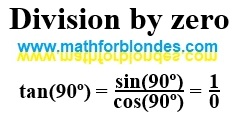Division by zero

The tangent of 90 degrees is equal to one divided by zero. That's what the math says. To understand division by zero, you need to know mathematics much better than we know it. If you firmly believe that zero is a number, you will never understand division by zero. Here you can find some of my publications on mathematics, which are somehow related to division by zero.

Division by zero can be found in various places in mathematics: the sine theorem, the equation of the straight line y=kx and so on. I suggest you explore these formulas on your own. Write about your results in the comments. If necessary, I will show you under what conditions division by zero appears there.

What is division by zero, I will definitely tell you in the future. But before that, there will be many more interesting things.

## Trigonometry value table for engineers

For engineers, a trigonometry table of values should contain numbers so that calculations can be done quickly.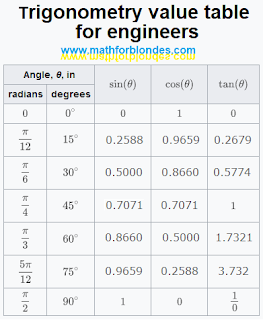Trigonometry value table for engineers

Division by zero is not as important for engineers as it is for physicists. Mathematicians have learned to bypass problem areas in engineering calculations. But physicists in their theories can meet division by zero much more often. If you already want to know what is the result of division by zero in your theoretical research, write in the comments, I will try to help you.